Preliminary results as of Summer 2014
(CKM 2014 conference)

 The global CKM fit: Inputs and Numerical results The global CKM fit: Pulls The global CKM fit in the large (ρ̅, η̅) plane The global CKM fit in the small (ρ̅, η̅) plane (zoom) The global CKM fit in the large (ρ̅M, η̅M) plane with M = sb, ds, ct, ut, uc Constraint from BR(B+→τ+ ν) New physics in neutral-meson mixing Branching ratio of Bs→μ+ μ- The global CKM fit in the (|Vud|,|Vus) plane The global CKM fit in the (|Vcd|,|Vcs) plane Constraints on the angle α/ϕ2 from charmless B decays Constraints on the angle γ/ϕ3 from B decays to charm Constraints on |sin(2β+γ)|

Numerical results:

 The results of the global CKM analysis include: Wolfenstein parameters, UT angles and sides, UTsangle and apex, CKM elements, theory parameters, rare branching fractions (B->lν, B->ll).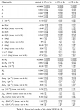Numerical Results

 Pulls for various inputs or parameters involved in the Standard Model global fit. Each pull (in units of σ) is computed by taking the square root of the difference between χ2min obtained including or not including direct information on the quantity. This corresponds to consider Δχ2X;min=χ2with data on X;min- χ2without data X;min as a random variable distributed with 1 degree of freedom, and reinterpret the probability of reaching the observed value in units of σ. The presence of a plateau in the Rfit model for theoretical uncertainties may lead to a vanishing pull for some quantities even in cases where the predicted and observed values are not identical. Many of the pulls presented in this plot are correlated [this is for instance the case for sin 2β and Br(B→τν)], so that the distribution of the corresponding p-values should not be expected to be flat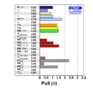eps png

Constraints in the (ρ̅, η̅) plane. The red hashed region of the global combination corresponds to 68% CL.
 α, β, γconvention ϕ1, ϕ2, ϕ3convention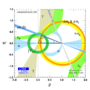eps png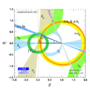eps png
Constraints in the (ρ̅, η̅) plane. The |Vub| constraint has been splitted in the two contributions: |Vub| from inclusive and exclusive semileptonic decays (plain dark green) and |Vub| from B+→τ+ ν (hashed green). The red hashed region of the global combination corresponds to 68% CL.
 α, β, γconvention ϕ1, ϕ2, ϕ3convention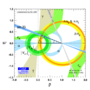eps png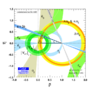eps png
Constraints in the (ρ̅, η̅) plane using only exclusive determinations of |Vub| and |Vcb| from semileptonic decays as inputs.
 α, β, γconvention ϕ1, ϕ2, ϕ3convention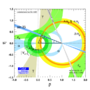eps png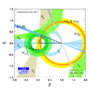eps png
Constraints in the (ρ̅, η̅) plane using only inclusive determinations of |Vub| and |Vcb| from semileptonic decays as inputs.
 α, β, γconvention ϕ1, ϕ2, ϕ3convention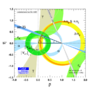eps png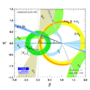eps png

Zoomed constraints in the (ρ̅, η̅) plane.The red hashed region of the global combination corresponds to 68% CL.
 α, β, γconvention ϕ1, ϕ2, ϕ3convention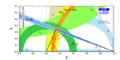eps png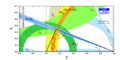eps png
Zoomed constraints in the (ρ̅, η̅) plane. The |Vub| constraint has been splitted in the two contributions: |Vub| from inclusive and exclusive semileptonic decays (plain dark green) and |Vub| from B+→τ+ ν (hashed green). The red hashed region of the global combination corresponds to 68% CL.
 α, β, γconvention ϕ1, ϕ2, ϕ3conventioneps png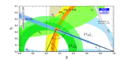eps png
Zoomed constraints in the (ρ̅, η̅) plane not including the angle measurements in the global fit.
 α, β, γconvention ϕ1, ϕ2, ϕ3convention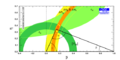eps png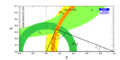eps png
Constraints in the (ρ̅, η̅) plane including only the angle measurements.
 α, β, γconvention ϕ1, ϕ2, ϕ3convention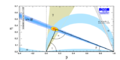eps pngeps png
Constraints from CP conserving quantities (|Vub / Vcb|, Δmd, (Δmd and Δms) and B+ →τ+ ν) in the (ρ̅, η̅) plane.
 α, β, γconvention ϕ1, ϕ2, ϕ3convention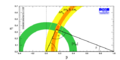eps png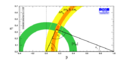eps png
Constraints from CP violating quantities (sin(2β), α, γ and εk) in the (ρ̅, η̅) plane.
 α, β, γconvention ϕ1, ϕ2, ϕ3convention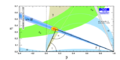eps png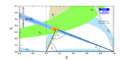eps png
Constraints from "Tree" quantities in the (ρ̅, η̅) plane (involving γ(DK) and α from the isospin analysis with the help of sin2β (charmonium), which gives another tree only γ measurement (the only assumption is that the ΔI=3/2 b-->d EW penguin amplitude is negligible)).
 α, β, γconvention ϕ1, ϕ2, ϕ3convention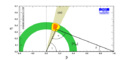eps png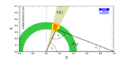eps png
Constraints from "Tree" quantities in the (ρ̅, η̅) plane (only γ(DK) is used).
 α, β, γconvention ϕ1, ϕ2, ϕ3convention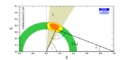eps png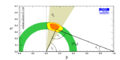eps png
Constraints from "Tree" quantities in the (ρ̅, η̅) plane with only input on |Vub| from semileptonic decays (ony γ(DK) is used).
 α, β, γconvention ϕ1, ϕ2, ϕ3convention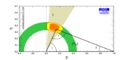eps png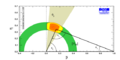eps png
Constraints from "Tree" quantities in the (ρ̅, η̅) plane with only input on |Vub| from exclusive semileptonic decays (only γ(DK) is used).
 α, β, γconvention ϕ1, ϕ2, ϕ3convention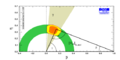eps png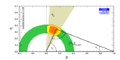eps png
Constraints from "Tree" quantities in the (ρ̅, η̅) plane with only input on |Vub| from inclusive semileptonic decays (only γ(DK) is used).
 α, β, γconvention ϕ1, ϕ2, ϕ3convention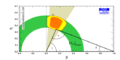eps png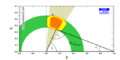eps png
Constraints from "Loop" quantities in the (ρ̅, η̅) plane.
 α, β, γconvention ϕ1, ϕ2, ϕ3convention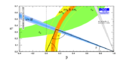eps png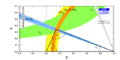eps png
Constraints in the (ρ̅, η̅) plane, not including the braching ratio of B+ → τ+ν in the global fit.
 α, β, γconvention ϕ1, ϕ2, ϕ3convention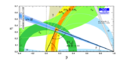eps png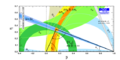eps png
Constraints in the (ρ̅, η̅) plane not including the measurement of sin2β in the global fit.
 α, β, γconvention ϕ1, ϕ2, ϕ3convention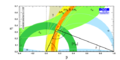eps png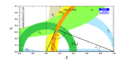eps png

The global CKM fit in the large (ρ̅M, η̅M) plane
with M = sb, ds, ct, ut, uc:

 The constraints can be expressed in the unitarity triangles associated with the different mesons of interest, with the relative coordinates of the upper appex of each triangle defined as ρ̅d1d2+iη̅d1d2 =-(Vud1Vud2*)/(Vcd1Vcd2*),     ρ̅u1u2+iη̅u1u2 =-(Vu1dVu2d*)/(Vu1sVu2s*).
Constraints in the (ρ̅sb, η̅sb) plane. The red hashed region of the global combination corresponds to 68% CL. The constraint from the angle φs related to Bs meson mixing is indicated with light blue lines.
 α, β, γconvention ϕ1, ϕ2, ϕ3convention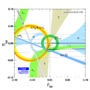eps png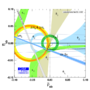eps png
Constraints in the (ρ̅ds, η̅ds) plane. The red hashed region of the global combination corresponds to 68% CL.
 α, β, γconvention ϕ1, ϕ2, ϕ3convention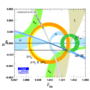eps png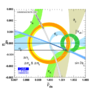eps png
Constraints in the (ρ̅tc, η̅tc) plane. The red hashed region of the global combination corresponds to 68% CL.
 α, β, γconvention ϕ1, ϕ2, ϕ3convention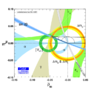eps pngeps png
Constraints in the (ρ̅tu, η̅tu) plane. The red hashed region of the global combination corresponds to 68% CL.
 α, β, γconvention ϕ1, ϕ2, ϕ3convention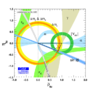eps png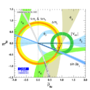eps png
Constraints in the (ρ̅cu, η̅cu) plane. The red hashed region of the global combination corresponds to 68% CL.
 α, β, γconvention ϕ1, ϕ2, ϕ3convention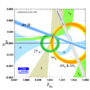eps png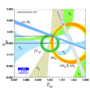eps png

 There is a specific correlation between sin2β and BR(B→τν), regarding the prediction from the global fit without using these measurements. The cross corresponds to the experimental value with 1 sigma errors. The discrepancy between prediction and measurement is 1.6 σ.eps png The constraint can also be seen from the point of view of lattice inputs, with the predictions on the decay constant fBd and fBd Sqrt[BBd]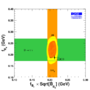eps pngIndividual constraints correspond to 68% CL (see: arXiv:1008.1593 [hep-ph] and arXiv:1203.0238. [hep-ph] for a detailed explanation of the hypotheses). The DO result for the dimuon asymmetry ASL has been recently corrected to take into SM contributions neglected beforehand (standard model CP violation in interference of decays with and without mixing of B<0\$ to flavour non-specific states), based on arXiv:1303.0175 [hep-ex]. This estimate was later criticised in Uli Nierste's talk at CKM14 as being too large a correction. In order to take into account an underestimation of the systematics attached to this estimate, we consider the results both with and without including ASL in the fit.
 In scenario I, we have introduced NP in M12q = M12SM,qΔq independently for Bd, Bs and K, corresponding to NP with arbitrary flavour structure. In the absence of ASL, constraints on New Physics in the (ReΔd,ImΔd) plane. A 0.9 σ deviation is obtained for the 2-dimensional SM hypothesis Δd = 1 (Re(Δd)=1, Im(Δd)=0).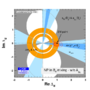eps png In the absence of ASL, constraints on New Physics in the (ReΔs,ImΔs) plane. A 0.3 σ deviation is obtained for the 2-dimensional SM hypothesis Δs = 1 (Re(Δs)=1, Im(Δs)=0). The pulls for the relevant observables are 1.1 σ for B->τν and 1.1 σ for φsJ/Ψ Φ.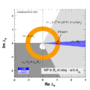eps png
 In the presence of ASL, constraints on New Physics in the (ReΔd,ImΔd) plane. A 1.2 σ deviation is obtained for the 2-dimensional SM hypothesis Δd = 1 (Re(Δd)=1, Im(Δd)=0).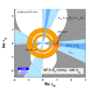eps png In the presence of ASL, constraints on New Physics in the (ReΔs,ImΔs) plane. A 0.3 σ deviation is obtained for the 2-dimensional SM hypothesis Δs = 1 (Re(Δs)=1, Im(Δs)=0). The pulls for the relevant observables are 0.2 σ for B->τν, 2.6 σ for φsJ/Ψ Φ and 2.4 σ for ASL.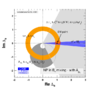eps png
 Prediction for Br(Bs→μ+ μ-)=(3.34+0.13-0.25)x10-9, to be compared with the current measurement (2.8+0.7-0.6)x10-9.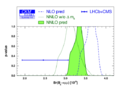eps png Prediction on the two dileptonic branching ratios Br(Bs→μ+ μ-) and Br(Bd→μ+ μ-) coming from the global fit (without input on dileptonic branching ratios) compared to current experimental information. In both plots, we consider the value of the branching ratios without time integration, which would induce a further increase of O(ΔΓs/Γs), more precisely (1+ys)=1.07.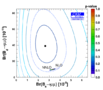eps png

 Constraints in the (|Vud|,|Vus|) plane. The indirect constraints (coming from b transitions) are related to |Vud| and |Vus| through unitarity. The red hashed region of the global combination corresponds to 68% CL.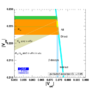eps png

 Constraints in the (|Vcd|,|Vcs|) plane. The indirect constraints (combing from b and s transitions) are related to |Vcd| and |Vcs| through unitarity. The direct constraints combine leptonic and semileptonic D and Ds decays as well as information from neutrino-nucleaon scattering and W → cs decays. The red hashed region of the global combination corresponds to 68% CL.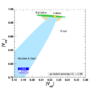eps png Constraints in the (|Vcd|,|Vcs|) plane where direct constraints involve only leptonic D and Ds decays with our inputs for lattice averages fDs=245.3 ± 0.5 ± 4.5 MeV and fDs/fD=1.201 ± 0.004 ± 0.010.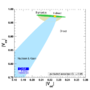eps png Constraints in the (|Vcd|,|Vcs|) plane where direct constraints involve only semileptonic D and Ds decays with our inputs for lattice averages FD → π(0)=0.666 ± 0.020 ± 0.048 and FD → K (0)=0.747 ± 0.011 ± 0.034.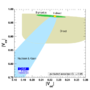eps png Constraints in the (|Vcd|,|Vcs|) plane where direct constraints involve only information from neutrino-nucleaon scattering and W→ cs decays (no lattice input).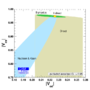eps png

Constraints on the angle α/ϕ2 from charmless B decays:

Constraints on α/ϕ2 from B→ππ, ρπ and ρρ (WA)
• α[WA,ππ]∈=(95.0+8.8-7.9
• α[WA,ρρ]=(89.9+5.4-5.3
• α[WA,all]=(87.7+3.5-3.3
compared to the prediction from the global CKM fit (not including the α-related measurements).
• α[fit]= (91.5+4.2-1.3
 α, β, γconvention ϕ1, ϕ2, ϕ3convention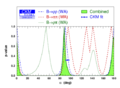eps png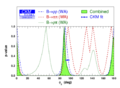eps png
Constraints on α/ϕ2 from the combination of B→ππ, ρπ, and ρρ for BaBar, Belle and WA, compared to the prediction from the global CKM fit (not including the α-related measurements).
 α, β, γconvention ϕ1, ϕ2, ϕ3convention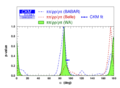eps png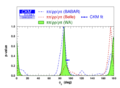eps png
Isospin triangles in B→ππ and B→ππ. One notices that the first triangle is flat, contrary to the second.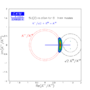eps png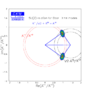eps png

Constraints on γ/ϕ3 from world average D(*)K(*) decays (GLW+ADS) and Dalitz analyses (GGSZ): γ[combined] = (73.2+6.3-7.0)°, compared to the prediction from the global CKM fit (not including these measurements):
γ[fit] = (66.9+1.0-3.7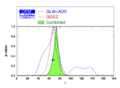eps png

Constraints on γ/ϕ3 from different set of measurements on D(*)K(*) decays (GLW+ADS) and Dalitz analyses (GGSZ) (methods shown separately):
• γ[BaBar] = (70 ± 18) °
• γ[Belle] = (73+13-15
• γ[LHCb] = (74.6+8.4-9.2
• γ[combined] = (73.2+6.3-7.0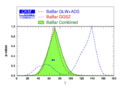eps png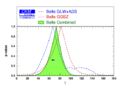eps png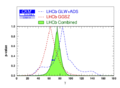eps png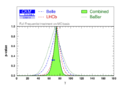eps png
Constraints on (rB(DK) vs γ) and (δB(DK) vs γ):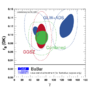eps png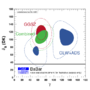eps png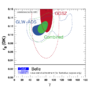eps pngeps png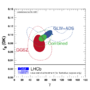eps png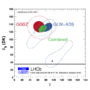eps png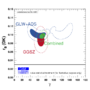eps pngeps png

 Constraint on the ratios of interfering amplitudes rB and the strong phases between the interfering amplitudes of the different decays B → D(*)K(*) using GLW, ADS and GGSZ results from these decays:
• rB(DK) = 0.0970+0.0062-0.0063,
• δB(DK) = (125.4+7.0-7.8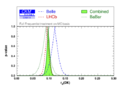eps png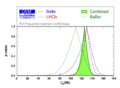eps png
• rB(D*K) =0.119+0.018-0.019,
• δB(D*K) = (-49+12-15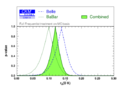eps png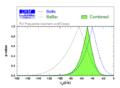eps png
• rB(DK*) = 0.137+0.051-0.047,
• δB(DK*) = (112+32-44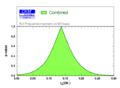eps png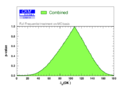eps png
• rB(DK*0) = 0.236+0.043-0.052,
• δB(DK*0) = (336+19-23)° and (200+10-9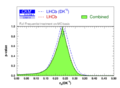eps png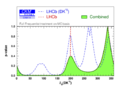eps png

 Constraints on |sin(2β+γ)| from the measurement of time-dependent CP asymmetries in D(*) π (ρ).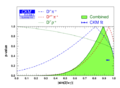eps png Translation of this result into γ (using sin(2β) as additional input and choosing among the four solutions to the SM one). γ[GLW+ADS+GGSZ+|sin(2β+γ)|] = (73.3+6.1-6.5)°.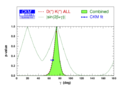eps png Constraints from |sin(2β+γ)| in the (ρ̅, η̅) plane.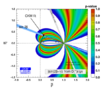eps png